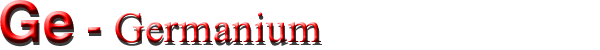## Band structure and carrier concentration

Basic Parameters
Temperature Dependences
Dependences on Hydrostatic Pressure
Energy Gap Narrowing at High Doping Levels
Effective Masses and Density of States
Donors and Acceptors

### Basic Parameters

 Energy gap 0.661 eV Energy separation (EΓ1) 0.8 eV Energy separation (ΔE>) 0.85 eV Energy spin-orbital splitting 0.29 eV Intrinsic carrier concentration 2.0·1013 cm-3 Intrinsic resistivity 46 Ω·cm Effective conduction band density of states 1.0·1019 cm-3 Effective valence band density of states 5.0·1018 cm-3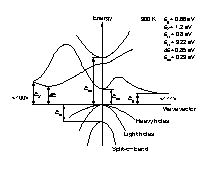Band structures of Ge.
 Eg= 0.66 eV Ex= 1.2 eV EΓ1 = 0.8 eV EΓ2 = 3.22 eV ΔE = 0.85 eV Eso = 0.29 eV

### Temperature Dependences

#### Temperature dependences of the energy gap:

Eg = 0.742- 4.8·10-4·T2/(T+235) (eV),
where T is temperature in degrees K.

#### Temperature dependence of the direct band gap EΓ1:

EΓ1 = 0.89 - 5.82·10-4·T2/(T+296) (eV),

#### Effective density of states in the conduction band:

Nc = 4.82·1015·M·[mc/mo]3/2·T3/2 (cm-3), or Nc = 1.98·1015·T3/2 (cm-3)
M = 4 is the number of equivalent valleys in the conduction band,
mc = 0.22mo is the effective mass of the density of states in one valley of the conduction band.

#### Effective density of states in the valence band:

Nν = 9.6·1014T3/2 (cm-3)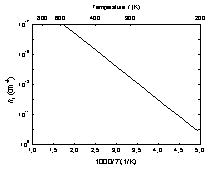The temperature dependence of the intrinsic carrier concentration ni.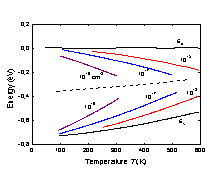Fermi level versus temperature for different concentrations of shallow donors and acceptors. Dashed line shows Fermi level dependence versus temperature for intrinsic Ge.

### Dependences on Hydrostatic Pressure

Eg = Eg(0) + 5.1·10-3P (eV)
EΓ1 = EΓ1(0) + 1.53·10-2P (eV),
where P is pressure in kbar.

### Energy Gap Narrowing at High Doping Levels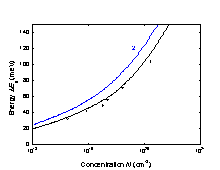Energy gap narrowing versus donor (curve 1) and acceptor (curve 2) doping density. Open curves are experimental values. (Jain and Roulston )

#### For n-type Ge:

ΔEg = 8.67·(Nd·10-18)1/3 + 8.14·(Nd·10-18)1/4 + 4.31·(Nd·10-18)1/2 (eV)

#### For p-type Ge:

ΔEg = 8.21·(Na·10-18)1/3 + 9.18·(Na·10-18)1/4 + 5.77·(Nd·10-18)1/2 (eV)
(Jain and Roulston ).

### Effective Masses

 Electrons: The surfaces of equal energy are ellipsoids ml = 1.59mo mt = 0.0815mo Effective mass of density of states mc=(9mlmt2)1/3 mc=0.22mo Effective mass of conductivity mcc=0.12mo Holes: Heavy mh = 0.33mo Light mlp = 0.043mo Split-off band mso = 0.084mo Effective mass of density of states mv = 0.34mo

### Donors and Acceptors

#### Ionization energies of shallow donors (eV):

 As P Sb Bi Li 0.014 0.013 0.010 0.013 0.093

#### Ionization energies of shallow acceptors (eV):

 Al B Ga In Tl 0.011 0.011 0.011 0.012 0.013